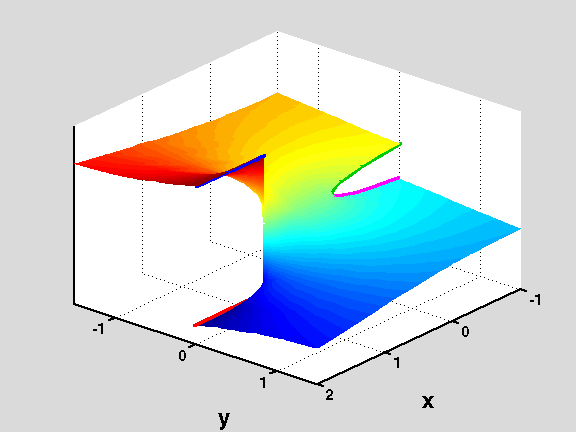18.04 | Fall 1999 | Undergraduate

# Complex Variables with Applications

Study Materials

## Riemann Surfaces: f(z)=log(1-z1/2) View 1

(All images created with MATLAB® software)

Surface generated by one of the branches where z=1 is a branch point of f(z)=log(1-z1/2). The other branches differ from this one by a multiple of 2πi and they give rise to infinitely many copies of this surface. To construct the Riemann Surface for f=f(z) we first stack (one on top of another) infinitely many copies of this surface.

Then we join them along the corresponding lips of the branch cuts on x > 1 (marked with thick red and blues lines on the figure) matching colors (red to blue on the next surface). This process leaves a gap at each level (due to the cut on x < 0, whose edges are marked by the magenta and green lines on the picture). These gaps are filled using the surfaces generated by the branches of f=f(z) that do not have z=1 as a branch point.

Picture obtained using the script RiemannSur in the Athena 18.04 MATLAB® Toolkit.Figure 1: Riemann Surface for the function f(z)=log(1-z1/2)

## Course Info

Fall 1999
##### Learning Resource Types
Exams with Solutions
Problem Sets with Solutions